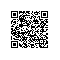# 躁动不安的年代，你需要读几本好书（python爬虫及数据分析）

1.书籍信息爬取

​ 1.1 requests 抓取网页

​ 1.2 BeautifulSoup ，re正则分析网页结构

2.信息分析

​ 2.1 pandas 处理文件

​ 2.2 pyecharts 可视化分析

### 1.网页抓取

1.常用方法

​ requests.get()
​ requests.post()
​ requests.put()
​ requests.delete()

2.参数

        method  提交方式（一般就是get ,post）
url     提交地址
params  GET请求中在URL中传递的参数，如http://xxxx?parameter=xxx 这种方式
data:    在请求体里传递的数据
json     在请求体里传递的数据
files    上传文件
timeout  请求和响应的超时时间
allow_redirects  是否允许重定向
proxies  代理
verify   是否忽略证书
cert     证书文件
stream   一部分一部分的获取数据，不用一次性获取数据，放入内存
session: 用于保存客户端历史访问信息


#伪装浏览器请求，User-Agent和Cookie 可以用你自己的，怎么取看下面的图
'User-Agent': '*******',
}

# print(res.status_code,res.apparent_encoding,res.content,res.encoding) #response响应的一些信息
res.encoding = res.apparent_encoding #设置请求头的编码
response = res.text #获取网页的内容### 2.分析网页，抓取数据

书名，链接，作者，出版社，出版日期，价格，评分，评论数，评论内容#用lxml方法来解析网页，默认是html.parse
soup = BeautifulSoup(response,'lxml')

#找到所有<li class = "subject-item">这样的标签，注意find_all方法返回的是list类型，下面使用的时候要用for循环，find是只找到第一个符合条件的标签，返回的是bs4.element类型，可以直接调用方法
artiche = soup.find_all('li','subject-item')#artiche是列表类型，循环操作每个元素
for item in artiche:
for i in item.find_all('div','info'): #书名和链接信息
try:
if i.find('a').string:  #防止存在没有书名的情况，string是获取a标签的内容
book_name = i.find('a').string.strip()  找到第一个a标签
else:
book_name='NULL'
if  i.find('a').attrs: #获取a标签的的属性
book_url = i.find('a').attrs.get('href').strip() #属性时字典方式，用get取数据
else:
book_url =url

pub_info = i.find('div','pub').string.strip() #出版社信息
book_info_list = pub_info.split('/')
#下面都是根据实际情况判断写的内容
if len(book_info_list)==5:
book_auth = book_info_list+','+book_info_list
book_publish = book_info_list
book_pub_date = book_info_list
book_price = re.findall('\d+',book_info_list)
elif len(book_info_list)==4:
book_auth = book_info_list
book_publish = book_info_list
book_pub_date = book_info_list
book_price = re.findall('\d+',book_info_list)
else:
book_auth = 'NULL'
book_publish = book_info_list
book_pub_date = book_info_list
book_price = re.findall('\d+', book_info_list)
#评分
rating_nums = i.find('span','rating_nums').string
#评论数信息
comment_nums = i.find('span','pl').string.strip()
comment_nums = re.findall('\d+',comment_nums)
comment_nums = comment_nums
#评论内容
if i.find('p'):
comment_content = i.find('p').string.strip().replace('\n','')
else:
comment_content= 'NULL'

print(book_name,
book_url,book_auth,book_publish,
book_pub_date,book_price,rating_nums,
comment_nums,comment_content)

               data_dict = {}
data_dict['书名'] = book_name
data_dict['链接'] = book_url
data_dict['作者'] = book_auth
data_dict['出版社'] = book_publish
data_dict['出版日期'] = book_pub_date
data_dict['价格'] = book_price
data_dict['评分'] = rating_nums
data_dict['评论数'] = comment_nums
data_dict['评论内容'] = comment_content

data_list.append(data_dict)

df = pandas.DataFrame(data_list_all)
df.to_csv('book.csv', encoding='utf_8_sig')  # encoding解决乱码问题

### 3.数据分析

from pyecharts import Bar,Pie
import pandas as pd

# print(df.loc[1:10,['书名','评论数']])
dfn = df.dropna(axis=0,subset=['书名'])  #删除书名为空的记录
# print(dfn['书名'],dfn['评论数'])
# print(dfn.loc[:,['书名','评论数']])

dfn_book_name = dfn_comment['书名'].values.tolist() #把dataframe类型转成list类型
dfn_comment_nums = dfn_comment['评论数'].values.tolist()

dfn_book_name_score = dfn_score['书名'].values.tolist()
dfn_comment_score = dfn_score['评分'].values.tolist()
# print(dfn_book_name,dfn_comment_nums,dfn_comment_score)
# print(type(df),type(dfn))
# print(dfn.dtypes['出版日期']) #打印列类型

#日期类型转换
# dfn['出版日期'] = pd.to_datetime(dfn['出版日期'],errors='coerce') #转换成日期类型
# dfn['出版日期'] = dfn['出版日期'].dt.year #取年份
dfn_pub_date = dfn
dfn_pub_date['出版日期'] = pd.to_datetime(dfn['出版日期'],errors='coerce') #转换成日期类型
dfn_pub_date['出版日期']= dfn['出版日期'].dt.year #取年份

# print(dfn_pub_date)
#根据出版日期年份分组，取出每年出版书籍数量
dfn_n = dfn_pub_date.groupby(['出版日期'],as_index=False)['书名'].size().reset_index(name='count')

#过滤出版数量在10以下的年份
dfn_n = dfn_n[dfn_n['count']>10]
dfn_n_year = dfn_n['出版日期'].values.tolist()
dfn_n_count = dfn_n['count'].values.tolist()

#最多产的出版社
dfn_n_pub = dfn.groupby(['出版社'],as_index=False)['书名'].size().reset_index(name='count')
dfn_n_pub_name = dfn_n_pub['出版社'].values.tolist()
dfn_n_pub_count = dfn_n_pub['count'].values.tolist()

1.根据评论数量和评分，分析大家对那些书敢兴趣，评分比较高bar = Bar("豆瓣文学类图书", "评价数量")
# bar.print_echarts_options() # 该行只为了打印配置项，方便调试时使用
bar.render('豆瓣文学评论数分析.html')  # 生成本地 HTML 文件
#

bar = Bar("豆瓣文学类图书", "评价数量")
# bar.print_echarts_options() # 该行只为了打印配置项，方便调试时使用
bar.render('豆瓣文学书籍评分分析.html')  # 生成本地 HTML 文件

2.各年份出版的书籍数量pie = Pie("各年份出版书籍数量分布饼图", title_pos='center')
label_text_color=None,
is_label_show=True,
legend_orient="vertical",
legend_pos="left")
# pie.show_config()
pie.render('年份出版书籍数量分布饼图.html')

3.各大出版的发行书籍数据占比pie = Pie("各出版社出版书籍数量分布饼图", title_pos='center')
label_text_color=None,
is_label_show=True,
legend_orient="vertical",
legend_pos="left")
# pie.show_config()
pie.render('各出版社出版书籍数量分布饼图.html')使用钉钉扫一扫加入圈子
+ 订阅